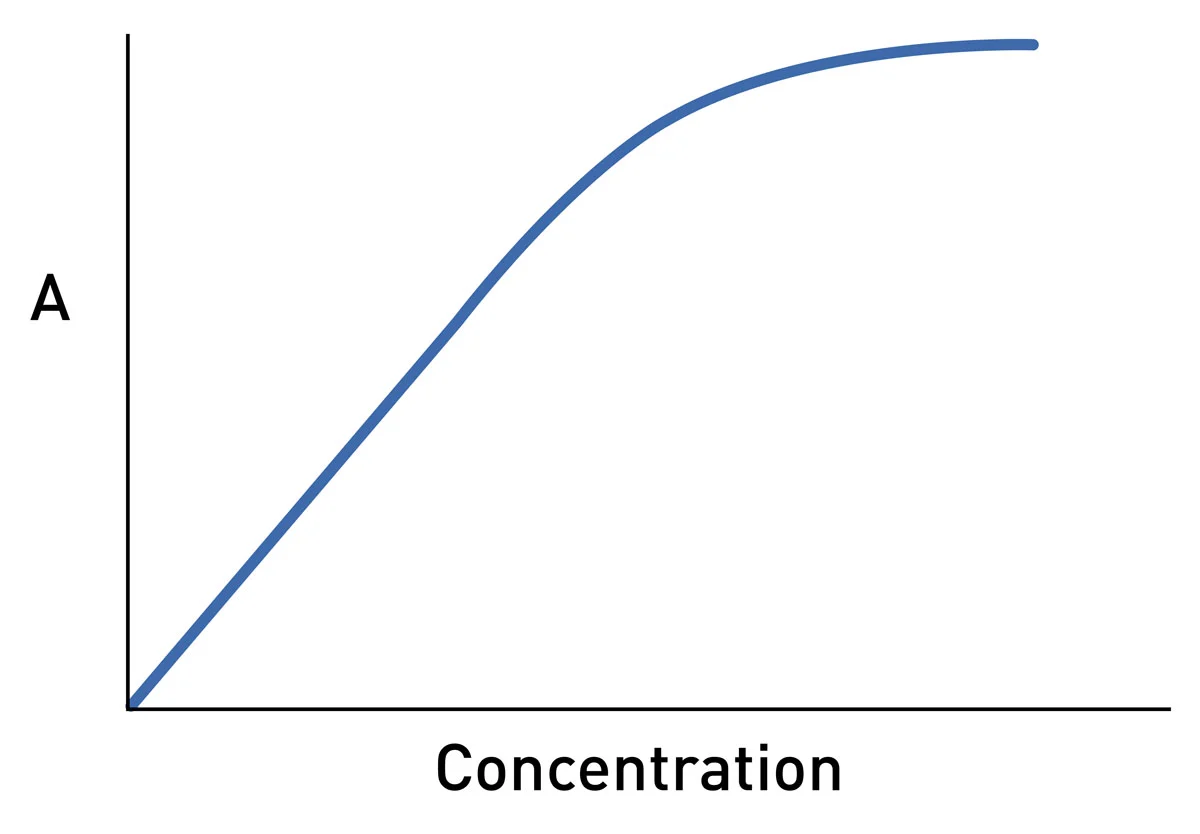# Optical Density for Absorbance Measurements

### For absorbance measurements, the optical density (O.D.) is a logarithmic measurement of the percent transmission (%T) and it can be represented by the equation, A = log10 100 / %T.

Here is a graphical scale that represents this:

That means a sample with:

• 1 O.D. allows 10% of light to be transmitted through the sample
• 2 O.D. allows 1% of light to be transmitted through the sample
• 3 O.D. allows 0.1% of light to be transmitted through the sample
• 4 O.D. allows 0.01% of light to be transmitted through the sample

In other words, if a sample has an O.D. greater than 3, this means that only 1 photon of light out of 1,000 will be measured by the detector. Even with the most sophisticated instruments, this small amount of light is very hard to accurately detect above the background noise. Therefore, measurements above 3 O.D. will have greater error and will in turn be less accurate than measurements taken at a lower O.D. Thus it is always recommended to dilute samples that are >3.0 O.D. and then to factor in the dilution factor to the final measurement. This is also shown in the following graph, note how the measurement is no longer linear at high concentrations, which correspond to higher O.D. values.